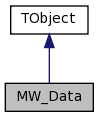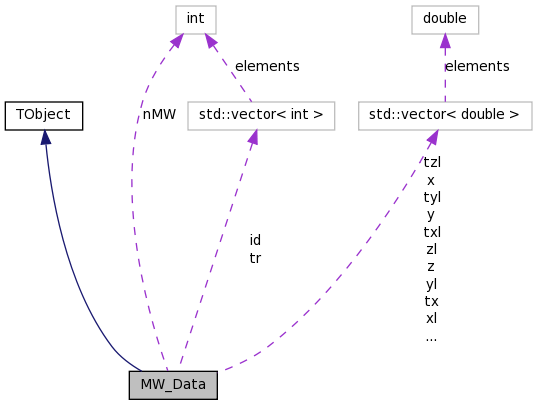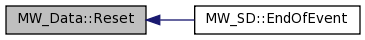# MW_Data Class Reference

MW_Data class. More...

`#include <MW_Data.h>`

Inheritance diagram for MW_Data:[legend]
Collaboration diagram for MW_Data:[legend]

List of all members.

## Public Member Functions

MW_Data ()
Constructor.
~MW_Data ()
Destructor.
void Reset ()
Clear the event vectors.

## Private Attributes

vector< double > e
vector< int > id
int nMW
vector< double > t
vector< int > tr
vector< double > tx
vector< double > txl
vector< double > ty
vector< double > tyl
vector< double > tz
vector< double > tzl
vector< double > x
vector< double > xl
vector< double > y
vector< double > yl
vector< double > z
vector< double > zl

class MW_SD

## Detailed Description

MW_Data class.

Defines the class used for writing data to the data file in the MW TBranch.

Definition at line 36 of file MW_Data.h.

## Constructor & Destructor Documentation

 MW_Data::MW_Data ( )

Constructor.

 MW_Data::~MW_Data ( )

Destructor.

Definition at line 36 of file MW_Data.cc.

## Member Function Documentation

 void MW_Data::Reset ( )

Clear the event vectors.

Definition at line 42 of file MW_Data.cc.

Referenced by MW_SD::EndOfEvent().

Here is the caller graph for this function:## Friends And Related Function Documentation

 friend class MW_SD` [friend]`

Definition at line 56 of file MW_Data.h.

## Member Data Documentation

 vector MW_Data::e` [private]`

Definition at line 63 of file MW_Data.h.

Referenced by MW_SD::EndOfEvent().

 vector MW_Data::id` [private]`

Definition at line 60 of file MW_Data.h.

Referenced by MW_SD::EndOfEvent().

 int MW_Data::nMW` [private]`

Definition at line 58 of file MW_Data.h.

Referenced by MW_SD::EndOfEvent().

 vector MW_Data::t` [private]`

Definition at line 64 of file MW_Data.h.

Referenced by MW_SD::EndOfEvent().

 vector MW_Data::tr` [private]`

Definition at line 61 of file MW_Data.h.

Referenced by MW_SD::EndOfEvent().

 vector MW_Data::tx` [private]`

Definition at line 66 of file MW_Data.h.

Referenced by MW_SD::EndOfEvent().

 vector MW_Data::txl` [private]`

Definition at line 70 of file MW_Data.h.

Referenced by MW_SD::EndOfEvent().

 vector MW_Data::ty` [private]`

Definition at line 67 of file MW_Data.h.

Referenced by MW_SD::EndOfEvent().

 vector MW_Data::tyl` [private]`

Definition at line 71 of file MW_Data.h.

Referenced by MW_SD::EndOfEvent().

 vector MW_Data::tz` [private]`

Definition at line 68 of file MW_Data.h.

Referenced by MW_SD::EndOfEvent().

 vector MW_Data::tzl` [private]`

Definition at line 72 of file MW_Data.h.

Referenced by MW_SD::EndOfEvent().

 vector MW_Data::x` [private]`

Definition at line 74 of file MW_Data.h.

Referenced by MW_SD::EndOfEvent().

 vector MW_Data::xl` [private]`

Definition at line 78 of file MW_Data.h.

Referenced by MW_SD::EndOfEvent().

 vector MW_Data::y` [private]`

Definition at line 75 of file MW_Data.h.

Referenced by MW_SD::EndOfEvent().

 vector MW_Data::yl` [private]`

Definition at line 79 of file MW_Data.h.

Referenced by MW_SD::EndOfEvent().

 vector MW_Data::z` [private]`

Definition at line 76 of file MW_Data.h.

Referenced by MW_SD::EndOfEvent().

 vector MW_Data::zl` [private]`

Definition at line 80 of file MW_Data.h.

Referenced by MW_SD::EndOfEvent().

The documentation for this class was generated from the following files: